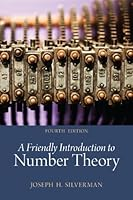# A Friendly Introduction to Number Theory, 4th Edition4 Reviews
2012-01-28
432 pages

## Book Description

A Friendly Introduction to , Fourth Edition is designed to introduce readers to the overall themes and methodology of mathematics through the detailed study of one particular facet—number theory. Starting with nothing more than basic high school , readers are gradually led to the point of actively performing research while getting a glimpse of current frontiers. The writing is appropriate for the undergraduate audience and includes many numerical examples, which are analyzed for and used to make conjectures. Emphasis is on the methods used for proving theorems rather than on specific results.

Chapter 1 What Is Number Theory?
Chapter 2 Pythagorean Triples
Chapter 3 Pythagorean Triplesand the Unit Circle
Chapter 4 Sums of Higher Powers and Fermat's Last Theorem
Chapter 5 Divisibility and the Greatest Common Divisor
Chapter 6 Linear Equations and the Greatest Common Divisor
Chapter 7 Factorization and the Fundamental Theoremof Arithmetic
Chapter 8 Congruences
Chapter 9 Congruences, Powers, and Fermat's Little Theorem
Chapter 10 Congruences, Powers, and Euler's Formula
Chapter 11 Euler's Phi Function and the Chinese Remainder Theorem
Chapter 12 Prime Numbers
Chapter 13 Counting Primes
Chapter 14 Mersenne Primes
Chapter 15 Mersenne Primes and Perfect Numbers
Chapter 16 Powers Modulo m and Successive Squaring
Chapter 17 kth Roots Modulo m
Chapter 18 Powers, Roots, and ''Unbreakable'' Codes
Chapter 19 Primality and Carmichael Numbers
Chapter 20 Squares Modulo p
Chapter 21 Is -1 a Square Modulo p? Is 2?
Chapter 23 Proof of Quadratic Reciprocity
Chapter 24 Which Primes Are Sums of Two Squares?
Chapter 25 Which Numbers Are Sums of Two Squares?
Chapter 26 As Easy as One, Two, Three
Chapter 27 Euler's Phi Function and Sums of Divisors
Chapter 28 Powers Modulo pand Primitive Roots
Chapter 29 Primitive Roots and Indices
Chapter 30 The Equation X4 + Y4 =Z4
Chapter 31 Square-Triangular Numbers Revisited
Chapter 32 Pell's Equation
Chapter 33 Diophantine Approximation
Chapter 34 Diophantine Approximation and Pell's Equation
Chapter 35 Number Theory and Imaginary Numbers
Chapter 36 The Gaussian Integers and Unique Factorization
Chapter 37 Irrational Numbers and Transcendental Numbers
Chapter 38 Binomial Coefficients and Pascal's Triangle
Chapter 39 Fibonacci's Rabbits and Linear Recurrence Sequences
Chapter 40 Oh, What a Beautiful Function
Chapter 41 Cubic Curves and Elliptic Curves
Chapter 42 Elliptic Curves with Few Rational Points
Chapter 43 Points on Elliptic Curves Modulo p
Chapter 44 Torsion Collections Modulo pand Bad Primes
Chapter 45 Defect Bounds and Modularity Patterns
Chapter 46 Elliptic Curves and Fermat's Last Theorem

## Book Details

• Title: A Friendly Introduction to Number Theory, 4th Edition
• Author:
• Length: 432 pages
• Edition: 4
• Language: English
• Publisher:
• Publication Date: 2012-01-28
• ISBN-10: 0321816196
• ISBN-13: 9780321816191